Miscellaneous

Chapter 7 Class 12 Integrals
Serial order wise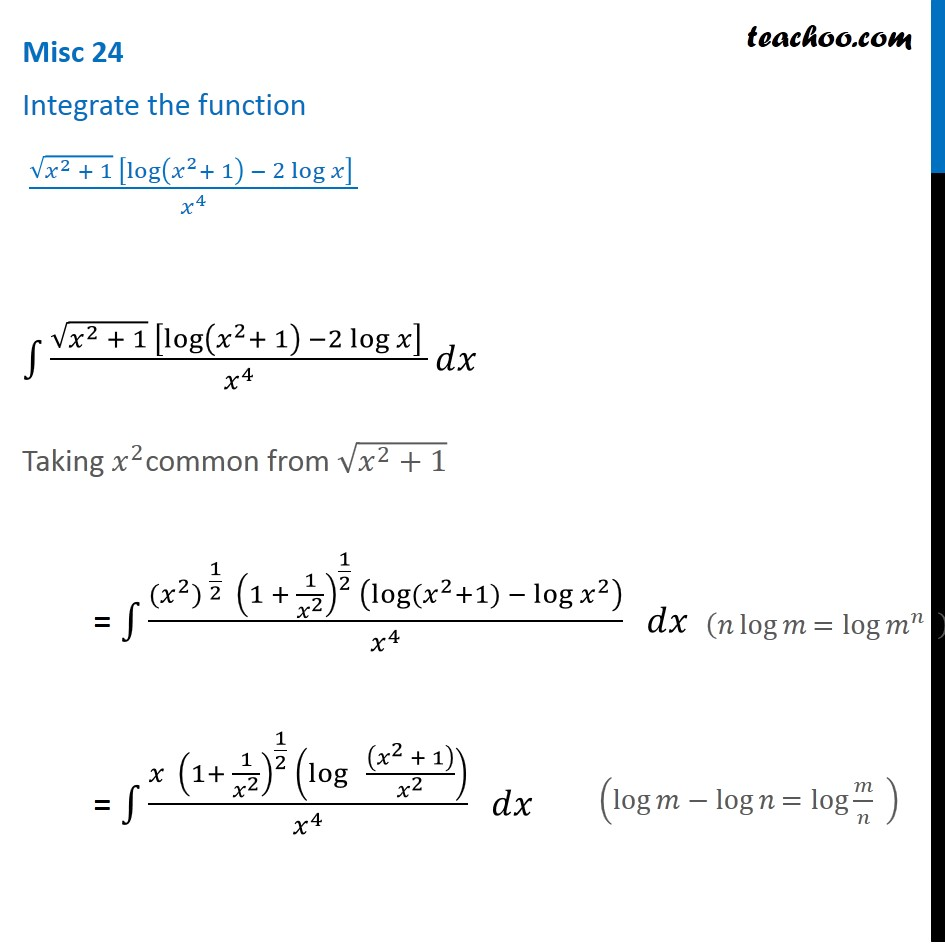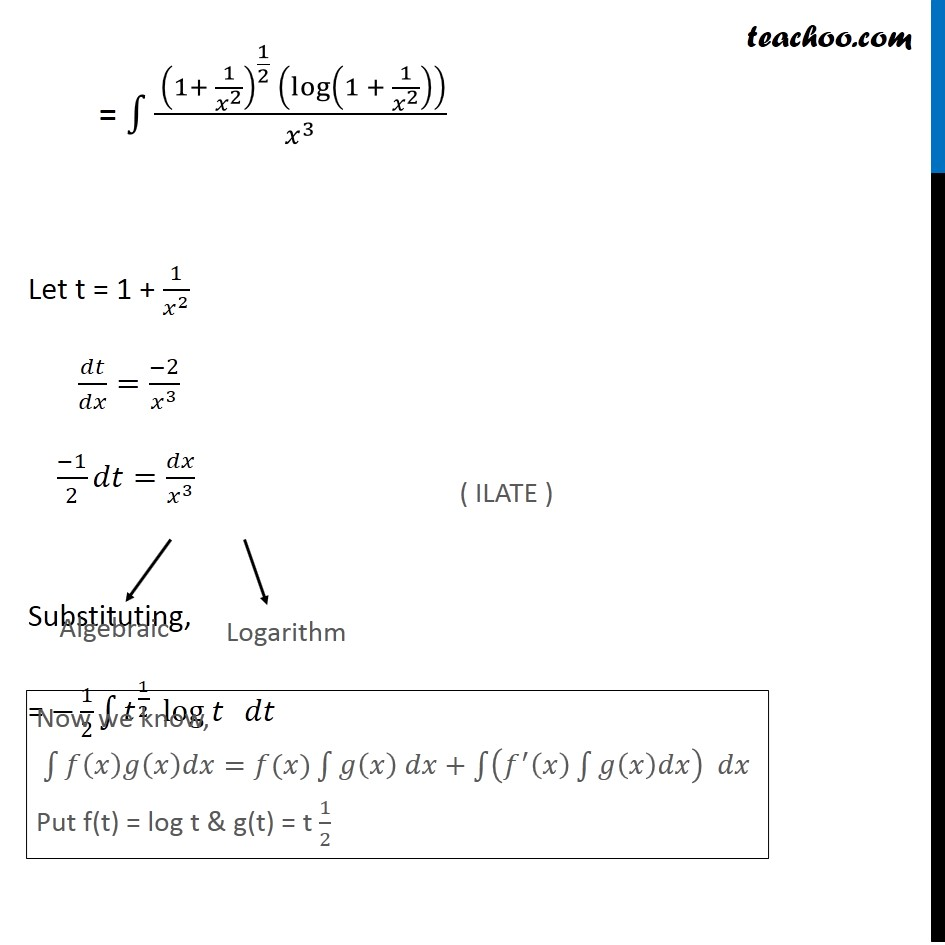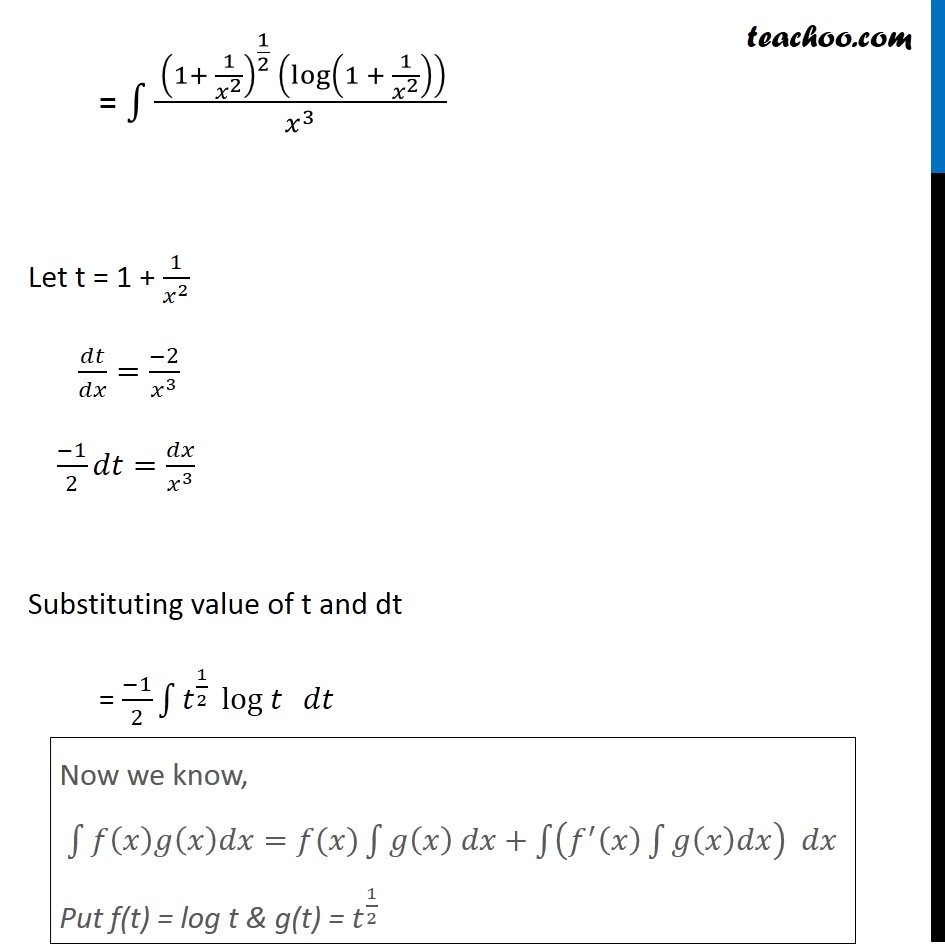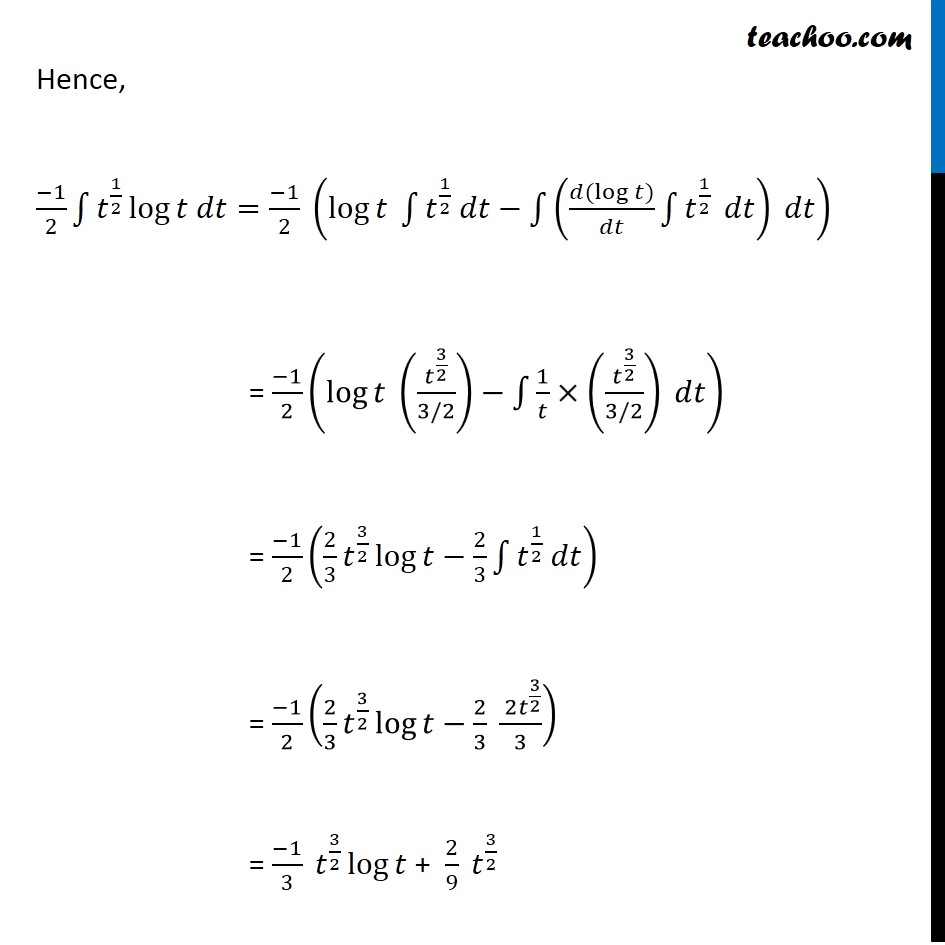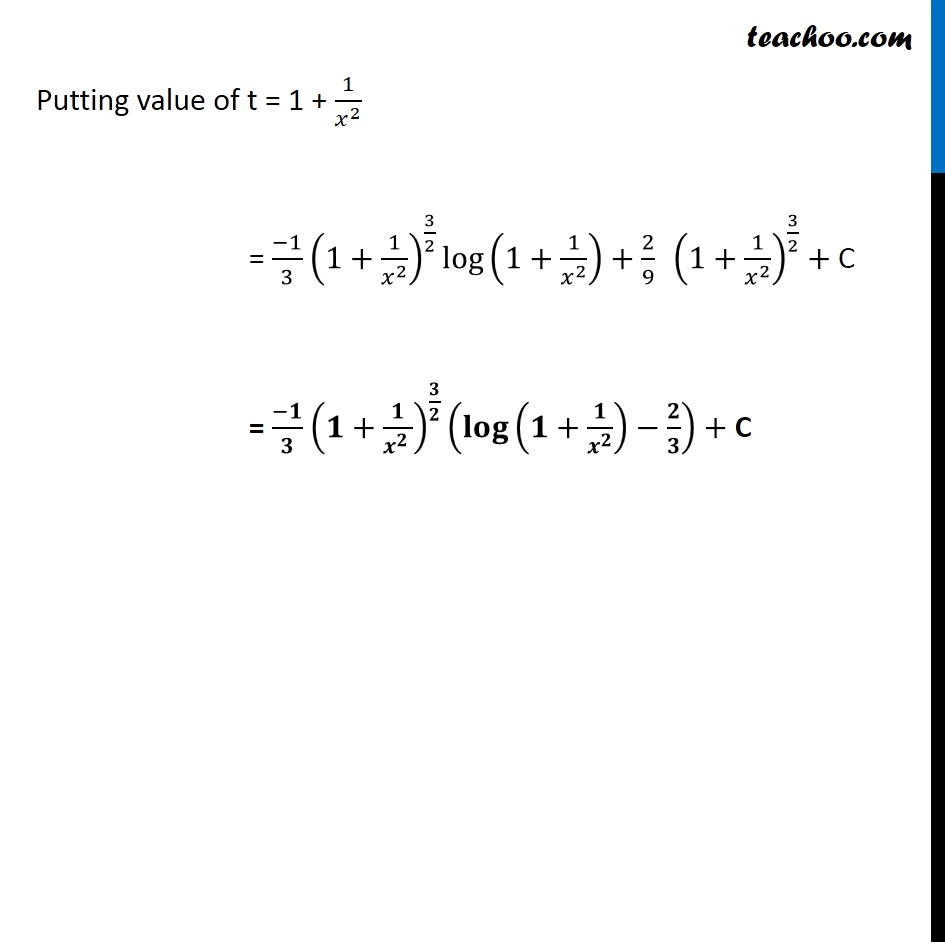Learn in your speed, with individual attention - Teachoo Maths 1-on-1 Class

### Transcript

Misc 23 Integrate the function (√(𝑥^2 + 1) [log⁡〖(𝑥^2+ 1) − 2 log⁡𝑥 〗 ] )/𝑥^4 ∫1▒(√(𝑥^2 + 1) [log⁡〖(𝑥^2+ 1) −2 log⁡𝑥 〗 ] )/𝑥^4 𝑑𝑥 Taking 𝑥^2common from √(𝑥^2+1) = ∫1▒(〖〖(𝑥〗^2) 〗^(1/2) (1 + 1/𝑥^2 )^(1/2) (log⁡〖(𝑥^2+1)〗 − log⁡〖𝑥^2 〗 ))/𝑥^4 𝑑𝑥 = ∫1▒(𝑥 (1+ 1/𝑥^2 )^(1/2) (log⁡〖 ((𝑥^(2 )+ 1))/𝑥^2 〗 ))/𝑥^4 𝑑𝑥 (𝑛 log⁡𝑚 〖=log〗⁡〖𝑚^𝑛 〗 " " ) (log⁡𝑚−log⁡𝑛 〖=log〗⁡〖𝑚/𝑛〗 " " ) = ∫1▒( (1+ 1/𝑥^2 )^(1/2) (log⁡(1 + 1/𝑥^2 ) ))/𝑥^3 Let t = 1 + 1/𝑥^2 𝑑𝑡/𝑑𝑥=(−2)/𝑥^3 (−1)/2 𝑑𝑡=𝑑𝑥/𝑥^3 Substituting, = −1/2 ∫1▒𝑡^(1/2) 〖 log 𝑡〗⁡〖 𝑑𝑡〗 Now we know, ∫1▒〖𝑓(𝑥)𝑔(𝑥)𝑑𝑥=𝑓(𝑥)∫1▒〖𝑔(𝑥) 𝑑𝑥+∫1▒〖(𝑓^′ (𝑥) ∫1▒𝑔(𝑥)𝑑𝑥) 𝑑𝑥〗〗〗 Put f(t) = log t & g(t) = t 1/2 = ∫1▒( (1+ 1/𝑥^2 )^(1/2) (log⁡(1 + 1/𝑥^2 ) ))/𝑥^3 Let t = 1 + 1/𝑥^2 𝑑𝑡/𝑑𝑥=(−2)/𝑥^3 (−1)/2 𝑑𝑡=𝑑𝑥/𝑥^3 Substituting value of t and dt = (−1)/2 ∫1▒𝑡^(1/2) 〖 log 𝑡〗⁡〖 𝑑𝑡〗 Now we know, ∫1▒〖𝑓(𝑥)𝑔(𝑥)𝑑𝑥=𝑓(𝑥)∫1▒〖𝑔(𝑥) 𝑑𝑥+∫1▒〖(𝑓^′ (𝑥) ∫1▒𝑔(𝑥)𝑑𝑥) 𝑑𝑥〗〗〗 Put f(t) = log t & g(t) = 𝑡^(1/2) Hence, (−1)/2 ∫1▒〖𝑡^(1/2) log⁡〖𝑡 𝑑𝑡=(−1)/2 (log⁡〖𝑡 ∫1▒〖𝑡^(1/2) 𝑑𝑡〗−∫1▒((𝑑(log⁡〖𝑡)〗)/𝑑𝑡 ∫1▒𝑡^(1/2) 𝑑𝑡) 𝑑𝑡〗 )〗 〗 = (−1)/2 (log⁡〖𝑡 (𝑡^(3/2)/(3/2))−∫1▒〖1/𝑡×(𝑡^(3/2)/(3/2)) 〗〗 𝑑𝑡) = (−1)/2 (2/3 𝑡^(3/2) log⁡〖𝑡−2/3〗 ∫1▒〖𝑡^(1/2) 𝑑𝑡〗) = (−1)/2 (2/3 𝑡^(3/2) log⁡〖𝑡−2/3〗 ( 〖2𝑡〗^(3/2))/3) = (−1)/3 𝑡^(3/2) log⁡𝑡 + 2/9 𝑡^(3/2) Putting value of t = 1 + 1/𝑥^2 = (−1)/3 (1+1/𝑥^2 )^(3/2) log⁡〖(1+1/𝑥^2 )+2/9 " " (1+1/𝑥^2 )^(3/2)+〗 C = (−𝟏)/𝟑 (𝟏+𝟏/𝒙^(𝟐 ) )^(𝟑/𝟐) (𝐥𝐨𝐠⁡(𝟏+𝟏/𝒙^𝟐 )−𝟐/𝟑)+ C